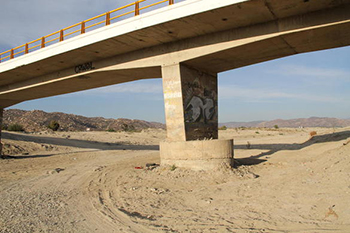online_scour_froehlich1988:  Calculation of pier scour using the Froehlich (1988) formulaFormula:

Fr1 = v1 / (g y1)1/2

ys = 0.32 b φ Fr10.2 (be / b)0.62 (y1 / b)0.46 (b / D50)0.08 + b

[ SI units (metric):  ys, y1, and b in m; v1 in m/s; D50 in mm ]
[ U.S. Customary units:  ys, y1, and b in ft; v1 in ft/s; D50 in mm ]
[ Fr = Froude number; g = gravitational acceleration ]

 SAMPLE INPUT      [Main page]     [Description] Flow depth directly upstream of the pier y1 =  1.0 m Mean flow velocity directly upstream of the pier v1 =  1.0 m/s Pier width in a horizontal direction normal to the flow b =  1.0 m Angle of attack of the flow θ =  0 degrees Median diameter of the bed material D50 =  1.0 mm Pier nose shape D50 :  Round nose SAMPLE OUTPUT               Scour depth ys =  1.4425 m